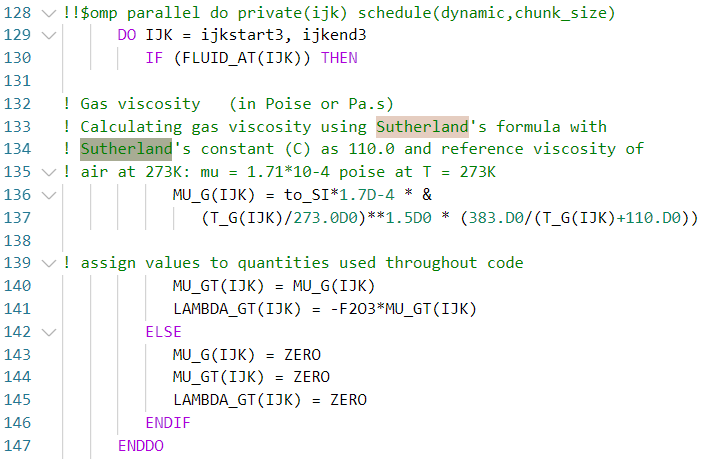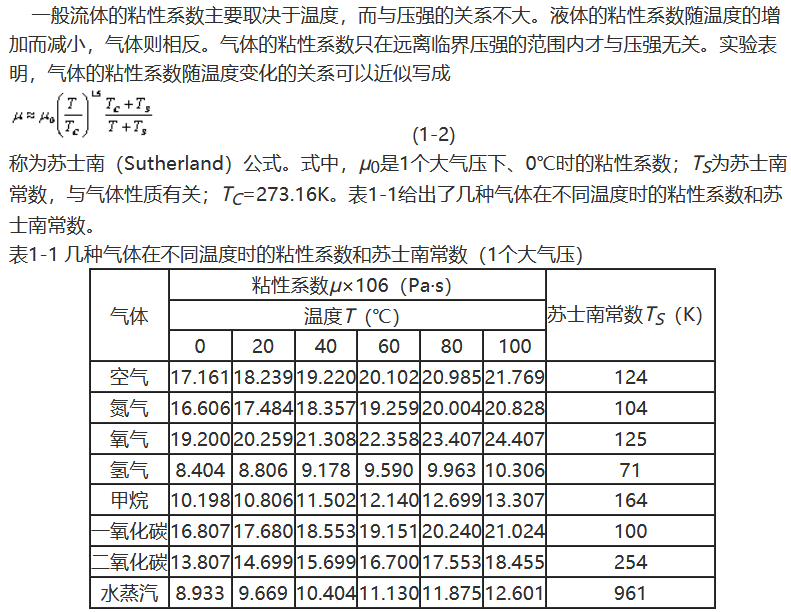• 动力粘度 题目 思路 计算 验证 毕业工作十多年，居然被别人问到高中的物理题。和日常工作有那么一丢丢关系。刚好好长时间没练手写markdown公式，勉为其难了。 题目 思路 关于动力粘度与内摩擦阻力之间，有如下公式...
欢迎关注，敬请点赞！
动力粘度题目思路计算验证
毕业工作十多年，居然被别人问到高中的物理题。和日常工作有那么一丢丢关系。刚好好长时间没练手写markdown公式，勉为其难了。
题目思路
关于动力粘度与内摩擦阻力之间，有如下公式：
$μ = \tau /(\frac{d_{u}}{d_{y}})$
$其中：\\μ——动力粘度；\\ \tau —— 单位面积内摩擦阻力；\\ \frac{d_{u}}{d_{y}}——为速度梯度。$
我是这么理解的，如果没有木板摩擦力带动，油膜可能是不动的，即相对于斜面的速度为0；
结果在木板带动下，靠近木板那一侧以速度v = 0.9 m/s运动。
速度梯度为速度差/距离。
那么：$\frac{d_{u}}{d_{y}} = \frac{v}{\delta} = \frac{0.9}{0.001} = 900\ s^{-1}$
另外：因木板匀速运动，重力斜向下的分力 = 摩檫力。
返回顶部
计算
则单位面积的内摩擦阻力为：$\tau = \frac{F}{A} = \frac{G×\sin 30^\circ}{0.4×0.6} = \frac{5×9.8×0.5}{0.24} = 10.21\ Pa$
结果：$μ = \tau /(\frac{d_{u}}{d_{y}}) = \frac{10.21}{900} = 0.0113 Pa·s = 11.3 \ mPa·s$
验证
日常生活中常见的动力粘度为：$水μ = 1.01×10^{-3}\ Pa·s\\甘油μ=1.499\ Pa·s$
所得结果的动力粘度在水和甘油之间，应该是对的。
欢迎关注，敬请点赞！
返回顶部


展开全文markdown
•matlab
• 分子动力学模拟lammps 粘度表面张力分析in文件分子动力学模拟lammps 粘度、表面张力分析in文件
• Sutherland’s law是关于动力粘度 μ\muμ 的经验关联式： μ(T)=T3/2(T0+ST+S) \mu(T)=T^{3 / 2}\left(\frac{T_{0}+S}{T+S}\right) μ(T)=T3/2(T+ST0​+S​) 其中T0=273.15KT_0=273.15KT0​=273.15K,S=110.4KS=...
Sutherland’s law是关于动力学粘度 $\mu$ 的经验关联式：
$\mu(T)=T^{3 / 2}\left(\frac{T_{0}+S}{T+S}\right)$
其中$T_0=273.15K$,$S=110.4K$为经验参数。1
更详细的内容可参考.

A sharp-interface immersed boundary method for moving objects in compressible viscous flows, Computers & Fluids. ↩︎


展开全文• MFiX采用sutherland公式计算粘度只能适用于空气，因为源代码里只给了空气 源代码位置\mfix-19.3.1\model\calc_mu_g.f ...动力粘度以273K 时 1.7E-4为基础，依照该网格温度变化 μg(IJK)=1.7×10−4×(Tg273)1.5×(3
MFiX采用sutherland公式计算粘度只能适用于空气，因为源代码里只给了空气
源代码位置\mfix-19.3.1\model\calc_mu_g.f其中F2O3为2/3
最主要的语句是136行
MU_G(IJK) = to_SI*1.7D-4 * &
(T_G(IJK)/273.0D0)**1.5D0 * (383.D0/(T_G(IJK)+110.D0))

动力粘度以273K 时 1.7E-4为基础，依照该网格温度变化
$\mu_g(IJK) = 1.7\times10^{-4} \times (\frac{T_g}{273})^{1.5}\times(\frac{383}{T_g+110})$
其中Tg是当地网格的气体温度
如果温度为1043K则计算出来为0.00042（4.2E-4）
可见温度的影响是十分明显的
不同气体的sutherland常数是不同的，MFiX中用的 是110
其他气体参考http://www.360doc.com/content/12/0216/14/492439_187081813.shtml展开全文• 假设发生热化，我们在相同的有效动力学理论框架（在此之前已计算出轻质部分贡献）的基础上，估计了对本体粘度的魅力贡献。 该物理学变得相关的时间尺度与与跟踪异常相关器相关联的传输峰的宽度有关，并且对于T≥600...
• 压缩包包含常见流体密度计运动粘度，动力粘度数据表，软件为通风管道阻力计算软件
• 涡旋粘度根据涡旋动力学以中尺度涡旋参数表示。 中尺度涡旋普查总体上显示，涡旋数在振幅，面积和寿命方面服从电子折叠衰减定律。 电子折叠定律中的内在值用于估算横向涡流粘度。 与先前的扩散率与长度平方成正比的...
• 第一章 计算流体力学动力学基础知识 CFD基本思想：把原来在时间域及空间域上连续的物理量场，如速度场和压力场，用一系列有限个离散点上的变量值的集合来代替，通过一定的原则和方式建立起关于这些离散点上场变量...

style: candy
第一章 计算流体力学动力学基础知识
CFD基本思想：把原来在时间域及空间域上连续的物理量场，如速度场和压力场，用一系列有限个离散点上的变量值的集合来代替，通过一定的原则和方式建立起关于这些离散点上场变量之间关系的代数方程组，然后通过解代数方程组获得场变量的近似解。
计算流体力学：通过计算机数值计算和图像显示的方法，在时间和空间上定量描述流场的数值解，从而达到对物理问题研究的目的。
CFD可以看做是在流动基本方程（质量守恒方程、动量守恒方程、能量守但方程）控制下对流动的数值模拟。通过这种数值模拟，我们可以得到极其复杂问题的流场内各个位置上的基本物理量（如速度、压力、温度、浓度等）的分布，以及这些物理量随时间的变化情况，确定旋涡分布特性、空化特性及脱流区等
计算流体动力学的工作步骤
采用CFD的方法对流体流动进行数值楼拟，通常包括如下步骤：
(1) 建立反映工程问题或物理问题本质的数学模型，，具体地说就是要建立反映问题各个量之间关系的微分方程及相应的定解条件，这是数值模拟的出发点。没有正确完善的数学模型，数值模拟就毫无意义。流体的基本控制方程通常包括质量守恒方程，动量守恒方程、能量守恒方程以及这些方程相应的定解条件，
(2) 寻求高效率、高准确度的计算方法，即建立针对控制方程的数值离散化方法．如有限差分法、有限元法、有限体积法等, 这里的计算方法不仅包括微分方程的离散化方法及求解方法，还包括贴体坐标的建立，边界条件的处理等。这些内容，可以说是CFD 的核心。
(3) 编制程序和进行计算这部分工作包括计算网格划分、初始条件和边界条件的输入、控制参数的设定等。这是整个工作中花时间最多的部分。由于求解的问题比较复杂，比如Navier-Stokes方程就是一个十分复杂的非线性方程，数值求解方法在理论上不是绝对完善的，所以需要通过实验加以验证。正是从这个意义上讲，数值模拟又叫数值试验。应该指出，这部分工作不是轻而易举就可以完成的。
(4) 显示计算结果。计算结果一般通过图表等方式显示，这对检查和判断分析质量和结果有重要参考意义。
有限差分法
将求解域划分为差分网格，用有限个网格节点代替连续的求解域，然后将偏微分方程的导数用差商代替，推导出含有离散点上有限个未知数的差分方程组。求出方程组的解，就是微分方程定解问题的数值近似解。
粘性
粘性(viscocity)是流体内部发生相对运动而引起的内部柜互作用
流体在静止时虽不能承受切应力，但在运动时，对相邻两层流体间的相对运动，即相对滑动速度却是有抵抗的，这种抵抗力称为粘性应力，流体所具有的这种抵抗两层流体间相对滑动速度，或普遍说来抵抗变形的性质，称为粘性.

注：计算流体力学的复杂性就在于粘性的复杂

流体热传导及扩散
除了粘性外，流体还有热传导及扩散(diffusion)等性质，当流体中存在着温度差时，温度高的地方将向温度低的地方传送热量．这种现象称为热传导。同样地，当流体混合物中存在着组元的浓度差时，浓度离的地方将向浓度低的地方输送该组元的物质，这种现象称为扩散。(温度不是产生热传导的唯一因素)

热传导定义：
热传导（thermal conduction）是介质内无宏观运动时依靠分子、原子及自由电子等微观粒子热运动而进行的传热传递现象，其在固体、液体和气体中均可发生，但严格而言，只有在固体中才是纯粹的热传导，而流体即使处于静止状态，其中也会由于温度梯度所造成的密度差而产生自然对流，因此，在流体中热对流与热传导同时发生。

流体的宏观性质，如扩散、粘性和热传导等，是分子输运性质的统计平均。由于分子的不规则运动，在各层流体间交换着质量、动量和能量，使不同流体层内的平均物理量均匀化。这种性质称为分子运动的输运性质。质量输运在宏观上表现为扩散现象，动量输运表现为粘性现象，能量输运则表现为热传导现象。
层流与湍流
自然界中的流体流动状态主要有两种形式，即层流(laminar)和湍流(turbulence) 。在许多中文文献中，湍流也被译为紊流。层流是指流体在流动过程中两层之间没有相互混掺，而湍流是指流体不是处于分层流动状态。—般说来，湍流是普遍的，而层流则属千个别情况。
流体动力学控制方程
流体流动要受物埋守恒定律的支配，基本的守恒定律包括：质最守恒定律、动量守恒定律、能量守恒定律。如果流动包含有不同成分（组元）的混合或相互作用．系统还要遵守组分守恒定律。如果流动处于湍流状态，系统还耍遵守附加的湍流输运方程。
控制方程(governing equations)是这些守恒定律的数学描述。本节先介绍这些基本的守恒定律所对应的控制方程．有关湍流的附加控制方程将在第4 章中介绍。
质量守恒方程
该定律可表述为：单位时间内流体微元体中质量的增加，等于同一时间间隔内流入该微元体的净质量。质量守恒方程也叫连续方程
$\frac{\partial\rho}{\partial t}+\frac{\partial(\rho u)}{\partial x} +\frac{\partial(\rho v)}{\partial y}+\frac{\partial(\rho w)}{\partial z}=0$
引入$div(\boldsymbol{a})=\partial a_x/\partial x+\partial a_y/\partial y+\partial a_z/\partial z$，用$\nabla$表示散度
KaTeX parse error: No such environment: gather at position 8:
\begin{̲g̲a̲t̲h̲e̲r̲}̲
\frac{\partial…
$u$为速度矢量，$u$,$v$,$w$是速度矢量$\boldsymbol{u}$在$x、y、z$方向的分量
对于不可压流体：
$\frac{\partial u}{\partial x} +\frac{\partial v}{\partial y}+\frac{\partial w}{\partial z}=0$
动量守恒方程
$\frac{\partial (\rho u)}{\partial t}+div(\rho u \mathbf{u})= - \frac{\partial p}{\partial x}+\frac{\partial \tau_{xx}}{\partial x}+\frac{\partial \tau_{yx}}{\partial y}+\frac{\partial \tau_{zx}}{\partial z}+F_x\\ \frac{\partial (\rho v)}{\partial t}+div(\rho v \mathbf{u})= - \frac{\partial p}{\partial y}+\frac{\partial \tau_{xy}}{\partial x}+\frac{\partial \tau_{yy}}{\partial y}+\frac{\partial \tau_{zy}}{\partial z}+F_y\\ \frac{\partial (\rho w)}{\partial t}+div(\rho w \mathbf{u})=- \frac{\partial p}{\partial z}+\frac{\partial \tau_{xz}}{\partial x}+\frac{\partial \tau_{yz}}{\partial y}+\frac{\partial \tau_{zz}}{\partial z}+F_z$
式中，$p$是流体微元体上的压力；$\tau_{xx}$、$\tau_{xy}$、$\tau_{xz}$等是因分子粘性作用而产生的作用在微元体表面上的粘性应力$\tau$的分量；$F_x$、$F_y$、$F_z$是微元体bb的体力，若体力为重力，且z轴竖直向下，则$F_z=-\rho g$
上式是对任何类型的流体（包括非牛顿流体）均成立的动量守恒方程。对于牛顿流体，$\tau$与流体的变形率成比例：

这里可看出N-S 方程局限于牛顿流体

N-S方程基本假设

流体是连续的。这强调它不包含形成内部的空隙，例如，溶解的气体气泡，而且它不包含雾状粒子的聚合。
所有涉及到的场，全部是可微的，例如压强$P$，速度$v$，密度$ρ$，温度$Q$，等等。该方程从质量，动量守恒，和能量守恒的基本原理导出。对此，有时必须考虑一个有限的任意体积，称为控制体积，在其上这些原理很容易应用。该有限体积记为ω，而其表面记为∂ω。该控制体积可以在空间中固定，也可能随着流体运动。
利用广义牛顿内摩擦定律，可以得到下面式子，详细推导见N-S方程推导
KaTeX parse error: No such environment: align at position 8:
\begin{̲a̲l̲i̲g̲n̲}̲
\tau_{xx} & =2…
式中，$\mu$是动力粘度(dynamic viscosity),$\lambda$是第二粘度(second viscosity)，一般可取$\lambda$=-2/3，带入原方程，
$\frac{\partial \tau_{xx}}{\partial x}=\frac{\partial} {\partial x} \left(2\mu\frac{\partial u}{\partial x}+\lambda div(\boldsymbol{u})\right) \\ \frac{\partial \tau_{yx}}{\partial y} = \frac{\partial } {\partial y}\left(\mu(\frac{\partial u}{\partial y}+\frac{\partial v}{\partial x})\right)\\ \frac{\partial \tau_{zx}}{\partial z} = \frac{\partial}{\partial z}\left(\mu(\frac{\partial u}{\partial z}+\frac{\partial w}{\partial x})\right)$
合并同类项，
KaTeX parse error: No such environment: align at position 7: \begin{̲a̲l̲i̲g̲n̲}̲
&\frac {\parti…
得：
$\frac{\partial(\rho u)}{\partial t}+\text{div}(\rho u\boldsymbol{u})=\text{div}(\mu \,\text{grad}\,u)-\frac{\partial p}{\partial x}+S_u \\ \frac{\partial(\rho v)}{\partial t}+\text{div(}\rho v\boldsymbol{u})=\text{div}(\mu \,\text{grad}\,v)-\frac{\partial p}{\partial y}+S_v\\ \frac{\partial(\rho w)}{\partial t}+\text{div}(\rho w\boldsymbol{u})=\text{div}(\mu \,\text{grad}\,w)-\frac{\partial p}{\partial z}+S_w$
式中，$grad()=\frac{\partial{()}}{\partial x}+\frac{\partial{()}}{\partial y}+\frac{\partial{()}}{\partial w}$，符号$S_u,S_v,S_w$是动量守恒方程的广义源项，$S_u=F_x+s_x$,$S_v=F_y+s_y,$,$S_w=F_z+s_z,$,$s_x、s_y、s_z$表达式如下:

KaTeX parse error: No such environment: gather at position 8:
\begin{̲g̲a̲t̲h̲e̲r̲}̲
s_x=\frac{\par…
一般来讲,$s_x、s_y、s_z$是小量，对于粘性为常数的不可压流体,$s_x=s_y=s_z=0$，所以我们常见的就是用$F_i$来替代$S_i$
N-S 方程可以张开如下面所示：
KaTeX parse error: No such environment: align at position 7: \begin{̲a̲l̲i̲g̲n̲}̲
& \frac{\parti…
能量方程
流体的能量$E$ 通常是内能$i$、动能$K=\frac{1}{2}(u^2+v^2+w^2)$和势能$P$三项之和，我们可针对总能量$E$建立能量守恒方程。但是，这样得到的能量守恒方程井不是很好用，一般是从中扣除动能的变化．从而得到关于内能$i$的守恒方程.而我们知道，内能$i$与温度$T$之间存在一定关系，即$i=c_{\rho}T$ 其中$C_{\rho}$是比热容。这样，我们可得到以温度$T$为变量的能量守恒方程
$\frac{\partial(\rho T)}{\partial t}+div(\rho \boldsymbol{u}T)=div\left(\frac{k}{c_{\rho}}\text{grad}\,T\right)+S_T\tag{3}$
该式可以展开为：
$\frac{\partial(\rho T)}{\partial t}+\frac{\partial(\rho uT)}{\partial x}+ \frac{\partial(\rho vT)}{\partial y}+\frac{\partial(\rho wT)}{\partial z}\\= \frac{\partial}{\partial z}\left(\frac{k}{c_{\rho}}\frac{\partial T}{\partial x}\right) - \frac{\partial}{\partial y}\left(\frac{k}{c_{\rho}}\frac{\partial T}{\partial y}\right) + \frac{\partial}{\partial z}\left(\frac{k}{c_{\rho}}\frac{\partial T}{\partial z}\right) +S_T$
综合基本方程$(1)、(2.1)、(2.2)、(2.3)、(3)$发现有$u,v,w,p,T,\rho$六个未知数，还需要补充联系$p$和$\rho$的状态方程,方程才能封闭，引入气体方程，
$p=p(\rho,T)$
$p=\rho RT$

注：对于液体如何让方程封闭还不是很了解

需要说明的是．虽然能量方程(3)是流体流动与传热问题的基本控制方程，但对于不可压流动，若热交换量很小以至可以忽略时，可不考虑能量守恒方程。这样，只需要联立求解连续方程(1)及动量方程(2.1)(2.2)(2.3)
此外，还需要注意，方程(1.13)是针对牛顿流体得到出的，对于非牛顿流体，应使用另外形式的能量方程，详见文献
组分质量守恒方程
$\frac{\partial (\rho c)}{\partial t}+div(\rho \boldsymbol{u}c_s)=div\big(D_s\text{grad}(\rho c_S)\big)+S_s$
通用形式:
工程热物理的世界就是这样神奇，所有的方程其实都可以用一个式子来表示：
$\frac{\partial (\rho \phi)}{\partial t} +div(\rho \boldsymbol{u}\phi)=div(\Gamma\,\text{grad}\,\phi)+S\tag{4}$
该式可以展开为：
$\frac{\partial(\rho \phi)}{\partial t}+\frac{\partial(\rho u\phi)}{\partial x}+ \frac{\partial(\rho v\phi)}{\partial y}+\frac{\partial(\rho w\phi)}{\partial z}\\= \frac{\partial}{\partial z}\left(\Gamma\frac{\partial \phi}{\partial x}\right) - \frac{\partial}{\partial y}\left(\Gamma\frac{\partial \phi}{\partial y}\right) + \frac{\partial}{\partial z}\left(\Gamma \frac{\partial \phi}{\partial z}\right) +S$
式中，$\phi$为通用变量，可以代表$u，v，w，T$，$\Gamma$为广义扩散系数;$S$为广义源项,上式各项分别为瞬态项、对流项、扩散项、源项。

方程
$\phi$
$\Gamma$
$S$

连续方程
1
0
0

动量方程
$u_i$
$\mu$
$\frac{\partial p}{\partial x_i}+S_i$

能量方程
T
$\frac{k}{T}$
$S_T$

组分方程
$c_s$
$D_{s}\rho$
$S_T$

湍流的能量方程
湍流是自然界非常普遍的流动类型，湍流运动的特征是在运动过程中液体质点具有不断的互相混掺的现象，速度和压力等物理量在空间和时间上均具有随机性质的脉动值
式(2)是三维瞬态Navier-Stokes方程，无论对层流还是湍流都是适用的。但对于湍流．如果直桵求解三维瞬态的控制方程．需要采用对计算机内存和速度要求很高的直接模拟方法，但目前还不可能在实际工程中采用此方法。工程中广为采用的方法是对瞬态Navier-Stokes 方程做时间平均处理，同时补充反映湍流特性的其他方程，如湍动能方程和湍流耗散率方程等。这些附加的方程也可以纳入式(4) 的形式中干采用同—程序代码来求解，对此将在这里超链接(后面补上)
守恒型控制方程
定义：对流项均采用散度的形式，$div(\rho \boldsymbol{u}\phi)$，物理量都在微分符号内，优点可以保持物理量守恒的性质，在有限体积法可以方便的建立离散方程，例子式(4)
非守恒型控制方程
定义：瞬态项和对流项的物理量从微分符号中移出
KaTeX parse error: No such environment: align at position 8:
\begin{̲a̲l̲i̲g̲n̲}̲
& \phi\frac{\…
参考：
.王福军-计算流体动力学分析:CFD软件原理与应用


展开全文• 我们在弛豫时间近似中，在纯杨-米尔斯理论和QCD中用光和奇夸克动力学理论研究了剪切粘度对熵密度比的温度依赖性。 作为非限定相中的有效自由度，我们考虑具有夸克和胶子量子数以及色散关系的拟粒子激发，而这些关系...
• 1.流体粘度的概念与测量方法 （1）.什么是粘度 粘度是表示流体在流动时，流体内部发生内摩擦的物理量，是流体反抗形变的能力。 1.物理定义 国标中对粘度的定义【1】：在两个平行平面间受剪切的流体，单位接触表...传感器
• 一、“第一性原理材料模拟及催化反应计算” 1、密度泛函基础与Linux常用命令 2、构建材料模型、结构优化，收敛性测试 3、吸附与催化反应、电荷分析、表面催化反应计算、频率计算与零点能矫正 4、能带与态密度计算及...经验分享
• 根据Kubo公式，我们使用（3 + 1）-d Parton级联Boltzmann多部分散射方法来计算在磁场存在下超相对论性Boltzmann气体的各向异性输运系数（剪切粘度和电导率）。 将结果与最近使用Grad近似获得的结果进行比较。 我们在...
• 关于举办“分子动力学模拟及第一性原理计算方法与应用”系列培训班的通知 各有关单位: 分子动力学模拟、第一性原理计算被广泛用于生命科学、化学工程、物理、医药、材料科学、电子学等领域。由于传统实验需要大量...
• 什么是粘度？ 通俗的讲，粘度就是表示流体在流动时，流体内部发生内摩擦的物理量，是流体反抗形变的能力。 ➀粘度的定义 ✎国标中对粘度的定义【1】：在两个平行平面间受剪切的流体，单位接触表面积上法向梯度为1...传感器
• 假设磁场是弱的或柔和的：eB〜g4log（1 / g）T2，我们以扰动QCD的对数为先导顺序计算了两种风味的QCD等离子体在外部磁场中的剪切粘度。 我们假设磁场是均匀且静态的，并且电动力学在形式极限e→0时是非动力学的，而...
• 通过使用此函数，您可以计算海水的以下特性作为温度和盐度的函数： 密度-焓-恒压比热-热导率-动力粘度-沸点升高matlab
• 在零粘度极限下，全息流体仍会产生熵，而动量扩散和声衰减在流体动力膨胀中的所有阶次都受到抑制。 通过将高阶导数电磁场项添加到该动作中，我们还计算了电荷扩散的校正量，并确定了电荷扩散常数消失的非微扰参数...
• 特定于患者的计算流体动力学在冠状动脉和心脏内血流模拟中的应用：挑战和机遇 心脏的新型心脏诊断和治疗方法的出现引起了针对特定患者环境的虚拟设计和技术测试领域的挑战。鉴于医学成像，计算能力和数学算法的最新...
• 得出：在计算低压下纯组分及混合组分气体的动力黏度时都比较精确，在计算高压下烃类及混合组分气体的动力黏度时拟合的新方程与API公式比较精确，高压下非烃类气体的动力黏度计算，API公式比较精确。但是，API公式...
• 基于计算流体动力学(CFD)分析软件Fluent,针对含纳米颗粒固液二相冲击射流，提出了一个新的基于等效粘度的多相流模型，并在不同纳米颗粒浓度下与不考虑固液相间相互作用的离散相模型(DPM)和考虑固液相间相互作用的DPM...
• 根据湿空气的相对湿度和温度计算空气参数（导热系数、密度、动力粘度、定压比热容、普朗特数）
• 对于两相区的动力粘度TP按下式计算。,(5-29),(6) 管长计算,(7) 质流量计算 在装置仿真中，毛细管的结构尺寸都是已知的，而需要求得的是流量等参数。其基本实现步骤如下：,在进口状态及出口背压已知条件下,先要确定...
• 实验28 甲烷部分氧化制合成气的催化剂制备、表征和性能 2．4 表面与胶体化学 实验29 液体粘度的测定 实验30 最大气泡法测定溶液表面张力 实验31 溶液吸附法测定比表面积 实验32 溶胶的制备与性质 实验33 粘度法测定...
• %参数计算: P=C*d, d是dimensionless无量纲值,P是physical参数，C是转化参数 %离散空间和时间尺度 δx和δt ,lb是离散数目. CC=P/lb是从物理参数到格子参数的转换参数lb。 deltas=1;deltat=1;lattc=deltas/delt...
• 循环水泵选型方法循环水泵选型的一般方法是根据水力计算的结果，得出地暖系统所需的水流量和克服地暖系统管网及壁挂炉本身阻力所需的扬程，综合考虑循环水泵在地暖系统中的工作效率，选择合适的循环水泵。...
• 我们计算状态方程，声波速度，传输系数（剪切和体积粘度），并讨论因果关系。 我们研究等离子体的均质和各向同性膨胀的流体力学级数的渐近特征。 我们对有限的c − a≠0进行计算，但是在保形对称破坏耦合中导致了不...
• 我们提出了相对论流体动力学方程，耦合了动态电磁场，包括极化，... 我们将“常规”磁流体动力学（使用物质中的麦克斯韦方程式计算）中的传输系数与“双”版本磁流体力学（使用守恒磁通量计算）中的传输系数联系起来。...